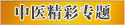# 古今中药剂量换算

《伤寒论》中剂量：1 斤 = 16 两 = 248 克= 液体250 毫升，1 两 = 24 铢 = 15.625 克。

《伤寒论》中剂量：

1 斤 = 16 两 = 248 克= 液体250 毫升

1 两 = 24 铢 = 15.625 克

1 圭 = 0.5 克

1 撮 = 2 克

1 方寸匕 = 金石类2.74 克= 药末约2 克= 草木类药末约1 克

1 斛 = 10 斗 = 20000 毫升

1 斗 = 10 升 = 2000 毫升

1 升 = 10 合 = 200 毫升

1 合 = 2 龠 = 20 毫升